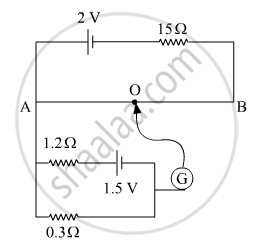# In the Following Potentiometer Circuit, AB is a Uniform Wire of Length 1 m and Resistance 10Ω. Calculate the Potential Gradient Along the Wire and Balance Length Ao (= L). - Physics

In the following potentiometer circuit, AB is a uniform wire of length 1 m and resistance 10 Ω. Calculate the potential gradient along the wire and balance length AO (= l).#### SolutionPotential space gradient = V_(AB)/I

Using  Kirchhoff’s rules,

2=Ixx15+Ixx10=Ixx25=>I=2/25=0.08 A=>V_(AB)=Ixx10=0.8 =V

therefore " Potential space gradient "=(0.8/1)=0.8V/m

Balance length = l

Potential difference across the balance length = Potential gradient × l

Potential difference across AO = 0.3 × 1 = 0.3 V

therefore 0.3 V="Potential space gradient" xxI=>0.3=0.8xxI=>I=0.375 m=37.5

Concept: Potential Difference and Emf of a Cell
Is there an error in this question or solution?